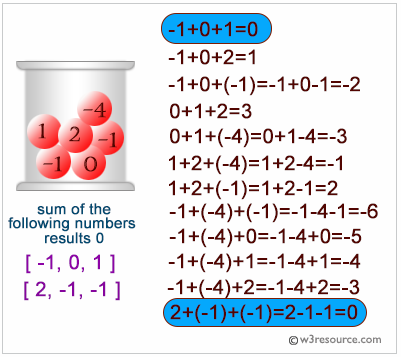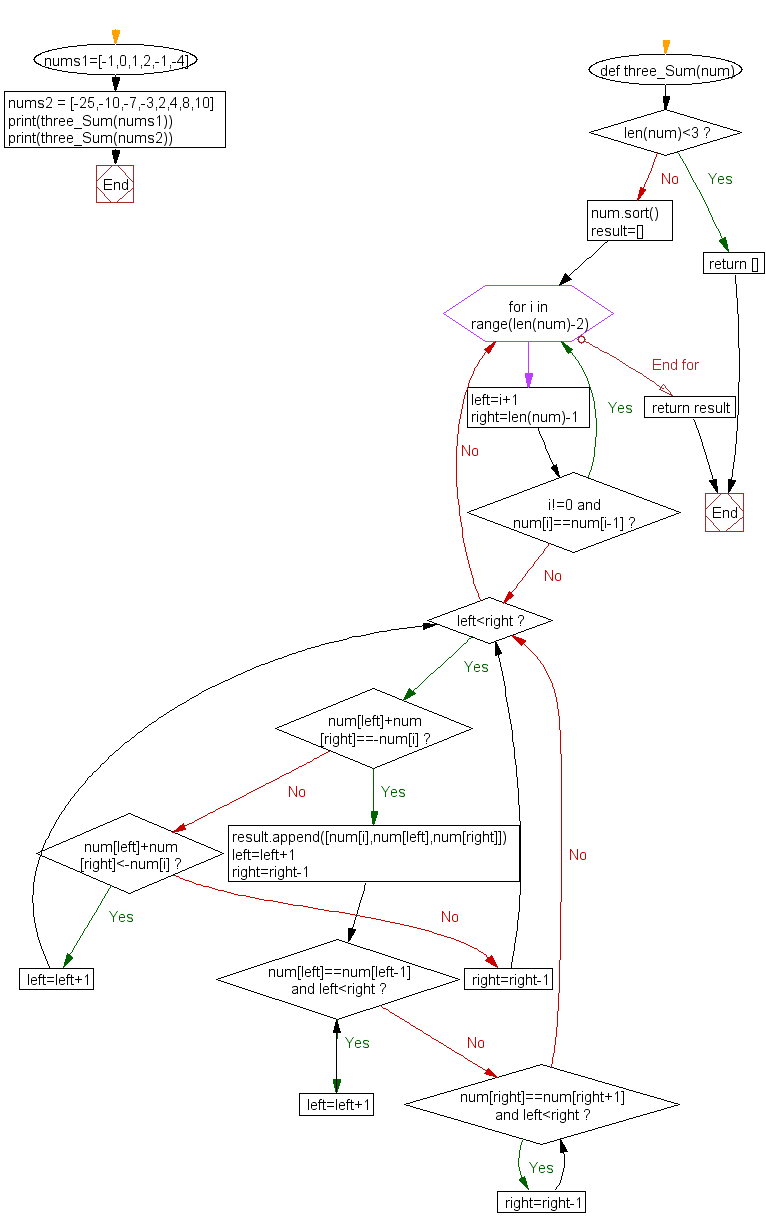﻿ Python: Find three numbers from an array such that the sum of three numbers equal to zero - w3resource# Python Challenges: Find three numbers from an array such that the sum of three numbers equal to zero

## Python Challenges - 1: Exercise-9 with Solution

Write a Python program to find three numbers from an array such that the sum of three numbers equal to zero.

Explanation:Sample Solution:-

Python Code:

``````# return a list of lists of length 3
def three_Sum(num):
if len(num)<3: return []
num.sort()
result=[]
for i in range(len(num)-2):
left=i+1
right=len(num)-1
if i!=0 and num[i]==num[i-1]:continue
while left<right:
if num[left]+num[right]==-num[i]:
result.append([num[i],num[left],num[right]])
left=left+1
right=right-1
while num[left]==num[left-1] and left<right:left=left+1
while num[right]==num[right+1] and left<right: right=right-1
elif num[left]+num[right]<-num[i]:
left=left+1
else:
right=right-1
return result

nums1=[-1,0,1,2,-1,-4]
nums2 = [-25,-10,-7,-3,2,4,8,10]
print(three_Sum(nums1))
print(three_Sum(nums2))
```
```

Sample Output:

```[[-1, -1, 2], [-1, 0, 1]]
[[-10, 2, 8], [-7, -3, 10]]
```

Flowchart:## Visualize Python code execution:

The following tool visualize what the computer is doing step-by-step as it executes the said program:

Python Code Editor:

What is the difficulty level of this exercise?

Test your Python skills with w3resource's quiz

﻿

## Python: Tips of the Day

Python: Kwargs

**kwargs and *args are function arguments that can be very useful.

They are quite underused and often under-understood as well.

Let's try to explain what kwargs are and how to use them.

• While *args are used to pass arguments at an unknown amount to functions, **kwargs are used to do the same but with named arguments.
• So, if *args is a list being passed as an argument, you can think of **kwargs as a dictionary that's being passed as an argument to a function.
• You can use arguments as you wish as long as you follow the correct order which is: arg1, arg2, *args, **kwargs. It's okay to use only one of those but you can't mix the order, for instance, you can't have: function(**kwargs, arg1), that'd be a major faux pas in Python.
• Another example: You can do function(*args,**kwargs) since it follows the correct order.
• Here is an example. Let's say satelites are given with their names and weight in tons in dictionary format. Code prints their weight as kilograms along with their names.
```def payloads(**kwargs):
for key, value in kwargs.items():
print( key+" |||", float(value)*100)
payloads(NavSat1 = '2.5', BaysatG2 = '4')
```

Output:

```NavSat1 ||| 250.0
BaysatG2 ||| 400.0```

Since the function above would work for any number of dictionary keys, **kwargs makes perfect sense rather than passing arguments with a fixed amount.

```def payloads(**kwargs):
for key, value in kwargs.items():
print( key+" |||", float(value)*100)

sats={"Tx211":"3", "V1":"0.50"}
```Tx211 ||| 300.0